# Abstracts

When experimental data are submitted to analysis of variance, the assumption of data homoscedasticity (variance homogeneity among treatments), associated to the adopted mathematical model must be satisfied. This verification is necessary to ensure the correct test for the analysis. In some cases, when data homoscedascity is not observed, errors may invalidate the analysis. An alternative to overcome this difficulty is the application of the specific residue analysis, which consists of the decomposition of the residual sum of squares in its components, in order to adequately test the correspondent orthogonal contrasts of interest between treatment means. Although the decomposition of the residual sum of squares is a seldom used procedure, it is useful for a better understanding of the residual mean square nature and to validate the tests to be applied. The objective of this review is to illustrate the specific residue application as a valid and adequate alternative to analyze data from experiments following completely randomized and randomized complete block designs in the presence of heteroscedasticity.

analysis of variance; completely randomized design; randomized complete block design

REVIEW

Specific residue: application of orthogonal contrasts when heteroscedasticity is present

Resíduo específico: aplicação de contrastes ortogonais na presença da heterocedasticidade

Maria Cristina Stolf Nogueira

USP/ESALQ - Depto. de Ciências Exatas, C.P.9 - 13418-900 - Piracicaba, SP - Brasil - e-mail <mcsnogue@esalq.usp.br>

ABSTRACT

When experimental data are submitted to analysis of variance, the assumption of data homoscedasticity (variance homogeneity among treatments), associated to the adopted mathematical model must be satisfied. This verification is necessary to ensure the correct test for the analysis. In some cases, when data homoscedascity is not observed, errors may invalidate the analysis. An alternative to overcome this difficulty is the application of the specific residue analysis, which consists of the decomposition of the residual sum of squares in its components, in order to adequately test the correspondent orthogonal contrasts of interest between treatment means. Although the decomposition of the residual sum of squares is a seldom used procedure, it is useful for a better understanding of the residual mean square nature and to validate the tests to be applied. The objective of this review is to illustrate the specific residue application as a valid and adequate alternative to analyze data from experiments following completely randomized and randomized complete block designs in the presence of heteroscedasticity.

Key words: analysis of variance, completely randomized design, randomized complete block design.

RESUMO

Introduction

The analysis of variance of experimental data requires that the assumption of homoscedasticity (similar variances among treatments), associated to the adopted mathematical model is satisfied. This verification is necessary for a correct significance of the test application. When this condition is not met the heteroscedasticity is prevailing (variance heterogeneity).

The heteroscedasticity can be classified as regular and irregular according to Steel and Torrie (1981) based on Cochran (1947). The regular type is generally originated from data non-normality and some type of relationship between means and variance treatments. In this case, the data may be transformed to have variance stability among treatments and, as a consequence, the errors will fit into an approximately normal distribution. The irregular type is characterized by certain treatments showing significantly higher variability compared to others, not necessarily presenting a relation between means and variances. In this case, Cochran and Cox (1957, 1971) recommended that such high variability treatments are omitted or that treatments are subdivided into homocedasticity groups in such way that they may present similar variances; or yet, to subdivide the residual sum of squares (SSResidual) in applicable components for the several comparisons of interest, thus obtaining specific residues.

When an analysis of variance is performed, the sum of squares of the treatments (SSTreatment) can be decomposed into components corresponding to orthogonal contrasts; in the same way, the residual sum of squares (SSResidual) can also be decomposed into their orthogonal contrast components, giving origin to the specific residues that are appropriate to test each contrast between treatment means.

The residual sum of squares (SSResidual) decomposition is not a usual procedure as the treatment sum of squares (SSTreatment) decomposition, but according to Cochran and Cox (1957, 1971), it can be applied when there are reasons suggesting the presence of irregular types of heteroscedasticity. In this case, the SSResidual decomposition is useful to better understand the residual mean square (MSResidual) nature and validate the tests to be applied.

A residual sum of squares (SSResidual) decomposition for experimental data of a randomized complete block design was presented by Steel and& Torrie (1981); initially, they established an orthogonal contrast grouping for treatments and thereafter they obtained the value of each contrast for each block. The authors concluded that if the randomized complete block design is valid, any comparison within each block is not influenced by the general level of the block. As a consequence, the variance for any comparison within blocks is appropriate to test contrasts between treatment means. The procedure was numerically shown.

In presence of the heteroscedasticity among experiments, when a group of experiments is considered, the interaction effects involving experiments (assumed as randomized effects) are influenced. An appropriate alternative to analyze the experimental data is the application of the specific residue method. With the objective to illustrate this case, Oliveira and Nogueira (2007) applied the specific residue method on sugarcane yield (t ha_1) experimental data obtained from a group of eleven experiments characterized by the presence of heteroscedasticity among experiments. Each experiment had a randomized incomplete block design, arranged in a 33 NPK factorial (27 treatments = three blocks ´ nine experimental units). The confounding of two degrees of freedom corresponding to the block effects plus NPK interaction effects was considered. No replication was applied to blocks.

The objective of this review is to illustrate the application of specific residues as an alternative procedure to analyze data showing heteroscedasticity among treatments.

Material and Methods

The methods, definitions and concepts on orthogonal contrasts applied to obtain specific residues can be found in Nogueira (2004). To bypassthe irregular heteroscedasticity present in the experimental data of a randomized complete block design, Ferreira (1978) presenteda mathematical procedure to obtain the specific residue sum of squares, correspondent to the appropriate components for comparisons (orthogonal contrasts) of interest, using the orthogonal transformation method. Thus, the specific residue sum of squares of the Yh component (SSR(Yh)) is given by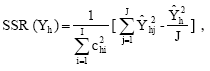with (J-1) degrees of freedom andis the Yhj contrast estimate, correspondent to the Yh contrast application within block j, for j = 1, ..., J,where I is the total number of treatments, for i = 1, ..., I; chi is the associated coefficient of the i-esimal treatment mean in the h-esimal contrast;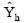is the h-esimal contrast estimate, for h = 1, ..., (I-1) ;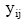is the observed value to i-esimal treatment in j-esimal block;the total sum of the i-esimal treatment and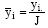the mean of the i-esimal treatment. Two contrasts are orthogonal when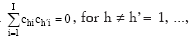for h ¹ h'= 1, ..., (I-1).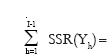SSR(Yh)=SSResidual has (I-1) (J-1) degrees of freedom and the residual mean square for Yh, MSR(Yh) =has (J-1) degrees of freedom.

Thus, the hypothesisfor h=1, ..., (I - 1), is tested by the application of the F test, and. where MS(Yh) is the mean square referred to the Yh component, with one degree of freedom, obtained as follows:In the case of a completely randomized design experiment in presence of irregular heteroscedascity SSResidual is decomposed in specific residues as shown by Nogueira (1984) and Nogueira and Campos (1985). These authors developed the decomposition of SSResidual and presented appropriate specific residues to test each contrast, and also identified how the specific residue sum of squares refers to the Yh component (SSR(Yh)). The development of the specific residue sum of squares in relationto the Yh component was obtained by applying the mathematical expectance (E) on SSR(Yh) of the randomized complete block design experiment, as follows: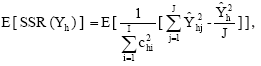assuming that E(ti) = ti, E(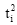) =, E(eij) = 0 and E(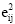)where ti is the i-esimal treatment effect, eij is the experimental error associated to yij. The specific residue sum of squares for Yh (SSR(Yh)) obtained is presented as follows:where SSTi is the i-esimal treatment sum of squares. Thus, the residual mean square for Yh (MSR(Yh)) is given by:with nh degrees of freedom, obtained by the application of the Satterthwaite (1941,1946) formula, and thus,and SSResidual =SSR(Yh) + SSR(among replications),

with I(J-1) degrees of freedom, and the SSR(among replications) is the residual sum of squares among replications, so thatwith (J-1) degrees of freedom and that residual mean square among replications (MSR(among replications) is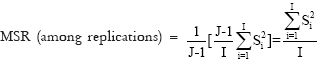Therefore, the hypotheses H0:Yh = 0 vs. Ha : Yh ¹ 0, for h=1, ..., (I - 1) were tested by the application of the F test, and the calculated F value was obtained through the expression:where MS(Yh) is the mean square of the Yh component, with one degree of freedom, obtained as follows:the followed the approximated F distributionswith one degree of freedom was referred to MS(Yh) with nh degrees of freedom obtained by the Satterthwaite (1941, 1946) formula and to MSR(Yh) as verified by Nogueira (1984). The verification was accomplished through the application of the simulation method developed by Godoi (1978), based on Box and Miller (1958), to variables with normal and one-dimensional distributions. The Chi-square test was applied to verify the adherence of Fh with the F(1,nh) distributions.

Results and Discussion

Completely randomized design

The experimental data shown in Table 1, cited by Nogueira (1984), refer to sorghum total dry matter yield, first cropping (g per pot) obtained from a completely randomized design experiment, with eight treatments and four replications, so that: Total for each treatmentwith (4 -1) degrees of freedom, where yij is the observed value (g per pot) of the i-esimal treatment in the j-esimal replication.

The variance for each treatment is given by, with (4-1) degrees of freedom and i = 1, ..., 8.

Preliminary analyses of variance results are presented in Table 2. Seven degrees of freedom for treatments and the sum of squares for treatments were decomposed according to the following group of orthogonal contrasts of interest: Y1: control treatments versus located and incorporated P-rates; Y2: among controls;Y3: Located versus incorporated P-rates; Y4: Linear effect of located P-rates; Y5: Quadratic effect of located P-rates; Y6: Linear effect of incorporated P-rates; Y7: Quadratic effect of incorporated P-rates.

Contrasts Y4 and Y5 provided the located-P treatment effect and contrasts Y6 and Y7, the incorporated-P treatment effect. The coefficients of applied contrasts and some results are shown in Table 3. As P-rates are not equidistant, the coefficients attributed to Y4, Y5, Y6 and Y7 contrasts were obtained using the orthogonal polynomial coefficient procedure for non-equidistant levels developed by Nogueira (1978) and cited by Nogueira (2007). The new analysis of variance with F test results without specific residue application is presented in Table 4.

If the model homoscedasticity assumption is satisfied, that is, if it is possible to consider that statisticallyMSResidual, the analysis presented in Table 4 is perfectly valid.

In order to verify the experimental data homoscedasticity, the Bartlett test was applied (among other tests), which is appropriate to test the following hypotheses: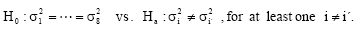The hypothesiswas rejected at p-value < 0.005 significance level, evidencing significant differences among variances due to the replications within treatments, characterizing the presence of heteroscedasticity. Once heteroscedasticity was evidenced, a procedure should be applied to overcome this situation. One alternative was the use of the specific residue as the F test denominator, to test each contrast defined in Table 3. This procedure consisted of the decomposition of all residual degrees of freedom (24), and consequently, the residual sum of squares obtaining the specific residue for each contrast: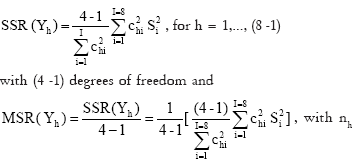degrees of freedom obtained through the application of the Satterthwaite (1941, 1946) formulaSSResidual =SSR(Yh) + SSR(among replications), with 8(4 -1) degrees of freedom. And SSR(among replications)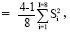, with (4 1) degrees of freedom and MSR (among replications)Thus, the hypothesisfor h=1, ..., (8 - 1), will be tested by the application of the F test and that, as observed by Nogueira (1984). Results are shown in Table 5, where the values in [ ], found in DF ( degrees of freedom) column refer to the effective degrees of freedom - nh , obtained by the Satterthwaite formula and applied in the F test.

It was observed that

MSResidual = MSR (among replications) =MSR (Yh) = 34.1734.

The F test values presented in Table 4 were obtained having MSResidual as denominator, with 24 degrees of freedom. The results presented in Tables 4 and 5 are different as well as some of the conclusions. This fact is important due to the presence of heteroscedasticity, because in Table 4, the MSResidual corresponds to the MSR(Yh) arithmetic mean; and in Table 5, the values obtained for MSR(Yh) were different. In the presence of homoscedasticity the values obtained for MSR(Yh) are very close to the ones obtained for MSResidual. The use of the specific residue procedure showed to be an interesting alternative to be applied when irregular heteroscedasticity is present, providing trustworthy results.

Randomized complete block design

In order to illustrate the specific residue procedure application on data analyses of a randomized complete block design experiment, the following experimental data were considered: yields of eight potato varieties (t ha-1) distributed in five blocks (Table 6).

The Bartlett test was applied to verify the variance homogeneity hypothesis, which was rejected, thus evidencing the presence of variance heterogeneity among treatments. Due to this fact and considering that experimental errors followed a normal distribution, the specific residue procedure was applied as an alternative for this data analysis. The initial analysis of variance is shown in Table 7.

Seven degrees of freedom and the variety sum of squares were decomposed in a group of orthogonal contrasts according to the high and low productivity criterion. Then, the potato varieties were divided into two groups and the high productivity potato group consisted of the varieties: (3) B1-52, (4) Huinkul, (5) B116-51; (6) B72-53 A and (7) S. Rafaela; and the low productivity potato group consisted of the varieties: (1) Kennebec, (2) B25-50E and (8) Buena Vista. Thus, the group of orthogonal contrasts built up according to the productivity criterion was: Y1: High productivity varieties (varieties 3, 4, 5, 6 and 7) versus Low productivity varieties (varieties 1, 2 and 8); Y2: Variety 7 versus varieties 3, 4, 5 and 6; Y3: Varieties 4 and 6 versus varieties 3 and 5; Y4: Between varieties 4 and 6; Y5: Between varieties 3 and 5; Y6: Variety 1 versus varieties 2 and 8; Y7: Between varieties 2 and 8.

The orthogonal contrasts Y2, Y3, Y4 and Y5 provided the high productivity variety effect with four degrees of freedom, and the contrasts Y6 and Y7 provided the low productivity variety effect with two degrees of freedom. The coefficients of the applied contrasts, the contrast estimates and the sum of squares obtained are shown in Table 8.

Twenty eight degrees of freedom and the residual sum of squares were decomposed according to the Y(h) components, resulting the Y(h) specific residues given by:with (5-1) = 4 degrees of freedom andis the Yhj contrast estimate, corresponding to the Yh contrast application in the block j, for j = 1, ..., J = 5 ,where yij is the observed value related to variety i in block j;is the h-esimal contrast estimate, for h = 1, ..., (8-1)=7 and. The values referred to yij and the Yh coefficients for thecalculus are presented in Table 9.

The results referred toandestimates and SSR(Yh) values are presented in Table 10, as follows:

It was observed thatSSR(Yh) = SQResidual = 348.324, with (8-1)(5-1)=28 degrees of freedom.

Also that MSR(Yh) =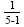SSR(Yh), with (5-1) = 4 degrees of freedom.

Thus, the hypotheses..., (8 - 1), were then tested by the application of the F test,The analysis of variance obtained with the specific residue procedure application is presented in Table 11. Significant F test values for Y1 and Y4 contrasts were observed, evidencing they differ from zero.

The analysis of variance without the specific residue procedure was also obtained (Table 12) in order to be compared to the previous analysis (Table 11). Significant F value was obtained for the Y1 contrast when calculated with MSResidual as denominator, with 28 degrees of freedom, evidencing that it significantly differed from zero. When the specific residue procedure was applied (Table 11), significant F values were obtained for the Y1 and Y4 contrasts.

Conclusion

The use of the specific residue procedure is a valid and efficient alternative when heteroscedasticity is present, because it validates the applied tests and also allows a better understanding of the residual mean square nature. The MSResidual corresponds to the MSR(Yh) arithmetic mean, although the values obtained for MSR(Yh) can be different. In the presence of homoscedasticity the values obtained for MSR(Yh) are very close to those obtained for MSResidual.

Accepted August 21, 2009

• Box, G.E.P; Miller, M.E. 1958. A note on the generation of random normal deviates. Annals of Mathematics Statistics 29: 610-611.
• Cochran, W.G. 1947. Some consequences when the assumptions for the analysis of variance are not satisfied. Biometrics 3: 2238.
• Cochran, W.G.; Cox, G.M. 1957. Experimental Designs. 2ed. John Wiley, New York, NY, USA.
• Cochran, W.G.; Cox, G.M. 1971. Diseños Experimentales. Editorial Trillas, Ciudad de México, México.
• Godoi, C.R.M. 1978. Um algoritmo eficiente para simulação de vetores com distribuição multinormal. Ciência e Cultura 20: 701-705.
• Ferreira, L.E.P. 1978. A decomposição do resíduo em casos de heterocedasticidade nas análises de variância de ensaios em blocos casualizados. MSc Dissertation. Universidade de São Paulo, Piracicaba, SP, Brazil. (in Portuguese with summary in English).
• Nogueira, I.R. 1978. Método geral para obtenção de tabelas de polinômios ortogonais. Revista da Agricultura 53: 269-279.
• Nogueira, M.C.S. 1984. Resíduo específico para contraste de tratamentos no delineamento inteiramente casualizado. Dr. Thesis. Universidade de São Paulo, Piracicaba, SP, Brazil (in Portuguese with summary in English).
• Nogueira, M.C.S.; Campos, H. 1985. Resíduo específico para contraste de tratamentos no delineamento inteiramente casualizado. Anais do Simpósio de Estatística Aplicada à Experimentação Agronômica 1. Fundação Cargill, Piracicaba, SP, Brazil.
• Nogueira, M.C.S. 2004. Orthogonal contrasts: Definitions and concepts. Scientia Agricola61: 118-124.
• Nogueira, M.C.S. 2007. Experimentação agronômica I. Conceitos, planejamento e análise de dados. Editora MCSNogueira, Piracicaba, SP, Brazil.
• Oliveira, W.; Nogueira, M.C.S. 2007. Aplicação do resíduo específico na análise de grupos de experimentos. Bragantia 66: 737-744.
• Satterthwaite, F.E. 1941. Synthesis of variance. Psychometrika 6: 309-316.
• Satterthwaite, F.E. 1946. An approximate distribution of estimates of variance components. Biometrics Bulletin 2: 110-114.
• Steel, R.G.D.; Torrie, J.H. 1981. Principles and Procedures of Statistics. 2ed. McGraw-Hill, New York, NY, USA.

# Publication Dates

• Publication in this collection
24 Mar 2010
• Date of issue
Feb 2010

• Accepted
21 Aug 2009